# Project 3b: Wave propagation in magnetized plasmas:

Imported and adapted from the UCLA Plasma Simulation Group's JupyterPIC Notebooks

Before anything else, we need to change to the stored notebook's directory, which contains input files for the PIC simulator.

cd /notebooks/x-mode-propagation

In this project, we are going to look at the propagation of an x-wave up a density gradient. The theory for high frequency waves propagating across a magnetic field in a plasma is in the notebook x-o-mode-theory. The goal of this project is for you to understand how the disperion relation can provide information regarding how a wave with a particular frequency behaves as it moves from vacuum into a magnetized plasma. You will study what happens with a wave starts with a frequency above the electron cyclotron frequency, $\omega_c$ but below the highest of the cut off frequencies, $\omega_R=\frac{1}{2}[\omega_c+(4\omega_{p0}^2+\omega_c^2)^{1/2}]$ where $\omega_{p0}$ is the plasma frequency at the highest density. You will launch waves into plasmas with either a sharp density rise or a gradual density rise. In both cases the electric field of the EM wave will be polarized in the $\hat x_2$ direction (the wave moves in the $\hat x_1$ direction and the applied magnetic field points in the $\hat x_3$ direction). You will also launch a wave with a frequency above $\omega_R=\frac{1}{2}[\omega_c+(4\omega_{p0}^2+\omega_c^2)^{1/2}]$ where the electric field of the wave is polarized in both the $\hat x_2$ and $\hat x_3$ directions (at an angle of $\pi/2$).

## 1. Plotting dispersion relations

The following several cells will plot relevant dispersion relations for magnetized plasmas near to what we will be simulating.

These are for waves in a plasma that has an external magnetic field such that $\Omega_{ce} = 0.5 \omega_{pe}$ and $\Omega_{ce} = 2.0 \omega_{pe}$.

# The following takes care of some initialization
from scipy.optimize import fsolve
import numpy as np
import matplotlib.pyplot as plt
%matplotlib inline

#first we define the range of k's of interest, "k" here means "ck"
karray=np.arange(0,2,0.05)
nk=karray.shape

def xwave_disp(w,omegap,omegac,ck):
ratio=omegac/omegap
y=(ck*ck)/(omegap*omegap)-(w*w)/(omegap*omegap)+(w*w/(omegap*omegap)-1)/(w*w/(omegap*omegap)-(1+ratio*ratio))
return y

warrayL=np.zeros(karray.shape); warrayR=np.zeros(karray.shape)
wLarray=np.zeros(karray.shape); wRarray=np.zeros(karray.shape)
wHarray=np.zeros(karray.shape)

### 1.1. Plotting dispersion relation for  $\Omega_{ce} = 0.5 \omega_{pe}$

#############
# here we specify the plasma conditions
wp=1
wc=0.5
#############

wR=0.5*(wc+np.sqrt(4*wp*wp+wc*wc))
wL=0.5*(np.sqrt(4*wp*wp+wc*wc)-wc)
wLarray[:]=wL
wRarray[:]=wR
wHarray[:]=np.sqrt(wp*wp+wc*wc)
warrayL=wL
warrayR=wR
for ik in range(1,nk):
warrayL[ik]=fsolve(xwave_disp,warrayL[ik-1],args=(wp,wc,karray[ik]))
warrayR[ik]=fsolve(xwave_disp,warrayR[ik-1],args=(wp,wc,karray[ik]))

plt.plot(karray,warrayR,'b',label='X wave ');   plt.plot(karray,warrayL,'b',label='X wave')
plt.plot(karray,wLarray,'--',label='$\omega_L$');   plt.plot(karray,wRarray,'--',label='$\omega_R$')
plt.plot(karray,wHarray,'--',label='$\omega_H$')
plt.xlabel('wave number $[ck/\omega_{pe}]$');   plt.ylabel('frequency $[\omega_{pe}]$')
plt.title('X wave dispersion relation,');   plt.legend()
plt.xlim([0,karray[nk-1]]);   plt.ylim([0,karray[nk-1]+1.0])
plt.legend(loc=0)
plt.show()print('w_L = '+repr(wL))
print('w_R = '+repr(wR))
print('w_H = '+repr(np.sqrt(wp*wp+wc*wc)))

### 1.2. Plotting dispersion relation for  $\Omega_{ce} = 2.0 \omega_{pe}$

#############
# here we specify the plasma conditions
wp=1
wc=2.0
#############

wR=0.5*(wc+np.sqrt(4*wp*wp+wc*wc))
wL=0.5*(np.sqrt(4*wp*wp+wc*wc)-wc)
wLarray[:]=wL
wRarray[:]=wR
wHarray[:]=np.sqrt(wp*wp+wc*wc)
warrayL=wL
warrayR=wR
for ik in range(1,nk):
warrayL[ik]=fsolve(xwave_disp,warrayL[ik-1],args=(wp,wc,karray[ik]))
warrayR[ik]=fsolve(xwave_disp,warrayR[ik-1],args=(wp,wc,karray[ik]))

plt.plot(karray,warrayR,'b',label='X wave ');   plt.plot(karray,warrayL,'b',label='X wave')
plt.plot(karray,wLarray,'--',label='$\omega_L$');   plt.plot(karray,wRarray,'--',label='$\omega_R$')
plt.plot(karray,wHarray,'--',label='$\omega_H$')
plt.xlabel('wave number $[ck/\omega_{pe}]$');   plt.ylabel('frequency $[\omega_{pe}]$')
plt.title('X wave dispersion relation,');   plt.legend()
plt.xlim([0,karray[nk-1]]);   plt.ylim([0,karray[nk-1]+1.0])
plt.legend(loc=0)
plt.show()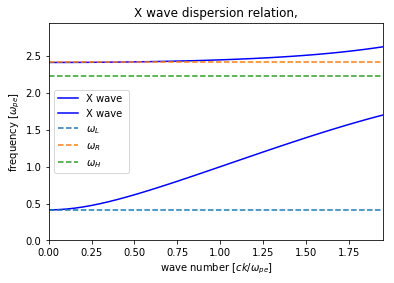print('w_L = '+repr(wL))
print('w_R = '+repr(wR))
print('w_H = '+repr(np.sqrt(wp*wp+wc*wc)))

## 2. Simulations with a Particle-in-Cell Code

The goal of this project is to study what happens when an electromagnetic wave is sent into a magnetized plasma in which the wave moves across the magnetic field.

You will simulate plasmas in which each plasma electron is initialized with positions (only in $x$ or what we call $x_1$) such that the plasma has a nonuniform density profile. The spacing between the electrons is proportional to the inverse of the density. Each electron is also initialized with velocities ($v_1$, $v_2$, $v_3$) = ($0.005c$, $0.005c$, $0.005c$) or momentum ($mv_1$, $mv_2$, $mv_3$) from a Maxwellian in each direction. The particles then begin to move in the self-consistent fields that their current and charge density produce. The peak density of the plasma in each case corresponds to $\omega_{p0}$=1.

For all cases, a constant magnetic field exists in the $x_3$ direction. It will be given a value corresponding to either $\omega_c/\omega_{p0}=0.5$ or $\omega_c/\omega_{p0}=2.0$.

At $t=0$, an electromagnetic (EM) wave is launched by an antenna from the left hand boundary along the $\hat x_1$ direction. The pulse grows in amplitude over $t=50\omega_{p0}^{-1}$ and then remains constant. In some cases the EM wave is polarized in the $E_2$ direction while in other cases it is polarized in the $E_2$ and $E_3$ directions at an angle of $\pi/2$.

### 2.1. The following lines must always be executed before running anything else.

Reminder: Hit Shift+Enter to run a cell, or select the cell and click on the "Run" button in the top menu bar

import osiris
%matplotlib inline

## 3. EM wave traveling up a density gradient;  $\omega_{0}/\omega_{p0} = 0.7$ ;  $\omega_{c}/\omega_{p0} = 0.5$.

• The length of the simulation window is $500 c/\omega_p$.
• There is no plasma between $x_1=0$ and $x_1=40 c/\omega_0$.
• The plasma density rises linearly from $x_1=40$ to $x_1=400 c/\omega_0$ to a value $n_0$ and the corresponding plasma frequency is $\omega_{p0}$.
• The density then remains constant at $n_0$ from $x_1=400$ to $x_1=500 c/\omega_0$.
• The simulation will run for a time 1100 $1/\omega_{p0}$.
• The simulation uses 50,000 particles.

You will launch a wave with its electric field pointing in the $x_2$ direction with $\omega_0=0.7 \omega_{p0}$. The peak value of the electric field will be $E_0=0.007 mc\omega_0/e$.

Run the simulation below:

# w0=0.7
dirname = 'xmode-b05-w07'
osiris.runosiris(rundir=dirname,inputfile='xmode-b05-w07.txt')

Plot the density:

dirname = 'xmode-b05-w07'
osiris.phasespace(rundir=dirname,dataset='x1',time=0,xlim=[0,500])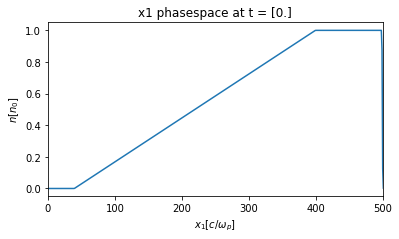You will next plot $E_2(t, x_1)$. The color corresponds to the amplitude of the EM wave. In addition, lines corresonding to the value of $x_1$ where the incoming EM wave's frequency $\omega_0$ equals $\omega_R$, $\omega_H$, $\omega_p$, and $\omega_L$ are also shown.

dirname = 'xmode-b05-w07'
osiris.plot_tx(rundir=dirname, b0_mag= 0.5, plot_or=2, w_0 = 0.7, n_peak = 1,one_0=40,one_D=360,
show_theory=True,cmap='seismic',xlim=[0,500])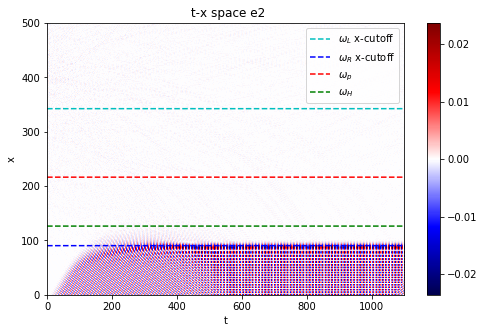• Did the wave start out with a frequency above or below $\omega_R$?
• The wave is reflected. How can you tell?
• At which cutoff is it reflected?

Next, let's zoom in a bit to see the behavior near the turning point:

dirname = 'xmode-b05-w07'
osiris.plot_tx(rundir=dirname, b0_mag= 0.5, plot_or=2, w_0 = 0.7, n_peak = 1,one_0=40,one_D=360,
show_theory=True,cmap='seismic',xlim=[0,200],tlim=[50,400])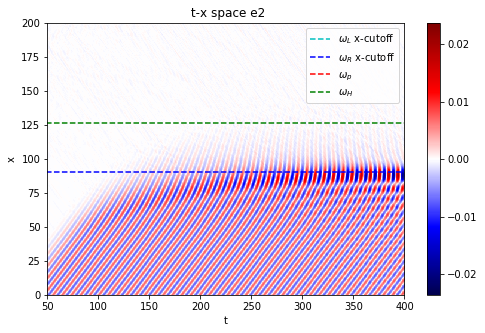• What is the phase and group velocity when it is reflected?
• Is this what you expect from the dispersion relation?

## 4. Light wave traveling up a density gradient;  $\omega_{0}/\omega_{p0} = 0.7$ ;  $\omega_{c}/\omega_{p0} = 2.0$.

This case is exactly the same as the first with the exception of the magnetic field amplitude.

• The length of the simulation window is $500 c/\omega_p$.
• There is no plasma between $x_1=0$ and $x_1=40 c/\omega_0$.
• The plasma density rises linearly from $x_1=40$ to $x_1=400 c/\omega_0$ to a value $n_0$ and the corresponding plasma frequency is $\omega_{p0}$.
• The density then remains constant at $n_0$ from $x_1=400$ to $x_1=500 c/\omega_0$.
• The simulation will run for a time 1100 $1/\omega_{p0}$.
• The simulation uses 50,000 particles.

You will launch a wave with its electric field pointing in the $x_2$ direction with $\omega_0=0.7 \omega_{p0}$. The peak value of the electric field will be $E_0=0.007 mc\omega_0/e$.

Run the simulation below:

# w0=0.7 w_p
dirname = 'xmode-b20-w07'
osiris.runosiris(rundir=dirname,inputfile='xmode-b20-w07.txt')

Plot the density:

dirname = 'xmode-b20-w07'
osiris.phasespace(rundir=dirname,dataset='x1',time=0,xlim=[0,500])Here we plot the wave pattern as it propagates up the density gradient:

dirname = 'xmode-b20-w07'
osiris.plot_tx(rundir=dirname, b0_mag= 2.0, plot_or=2, w_0 = 0.7, n_peak = 1, one_0=40,one_D=360, show_theory=True,cmap='RdBu')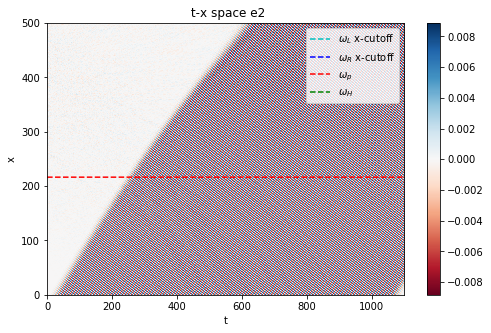• Did the wave start out with a frequency above or below $\omega_R$ when the density is 0?
• The wave is now transmitted. How can you tell?
• Can you explain why the wave does not get reflected in this case? (Hint: What is $\omega_L$ at the peak density?)

## 5. Light wave traveling up a density gradient;  $\omega_{0}/\omega_{p0} = 0.3$ ;  $\omega_{c}/\omega_{p0} = 2.0$.

The third case is the same as the second with the exception of the laser frequency and amplitude (the amplitude does not change the results).

• The length of the simulation window is $500 c/\omega_p$.
• There is no plasma between $x_1=0$ and $x_1=40 c/\omega_0$.
• The plasma density rises linearly from $x_1=40$ to $x_1=400 c/\omega_0$ to a value $n_0$ and the corresponding plasma frequency is $\omega_{p0}$.
• The density then remains constant at $n_0$ from $x_1=400$ to $x_1=500 c/\omega_0$.
• The simulation will run for a time 1100 $1/\omega_{p0}$.
• The simulation uses 50,000 particles.

You will launch a wave with its electric field pointing in the $x_2$ direction with $\omega_0=0.3 \omega_{p0}$. The peak value of the electric field will be $E_0=0.003 mc\omega_0/e$.

Run the simulation below:

# w0= 0.3 w_p
dirname = 'xmode-b20-w03'
osiris.runosiris(rundir=dirname,inputfile='xmode-b20-w03.txt')

Plot the density:

dirname = 'xmode-b20-w03'
osiris.phasespace(rundir=dirname,dataset='x1',time=0,xlim=[0,500])Next you will plot $E_2(t, x_1)$.

dirname = 'xmode-b20-w03'
osiris.plot_tx(rundir=dirname, b0_mag= 2.0, plot_or=2, w_0 = 0.3, n_peak = 1, one_0=40,one_D=360,show_theory=True,cmap='seismic')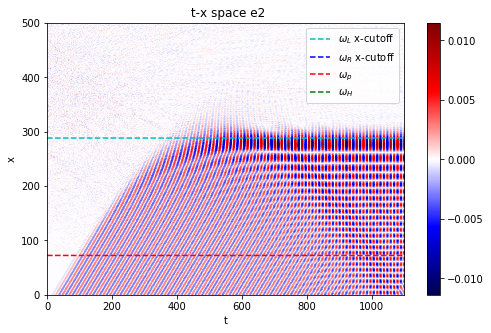• Why is the wave now reflected?
• Why is the wave now reflected at the density for which $\omega_0$=$\omega_L$?
• What is the phase and group velocity when it is reflected?

## 6. Light wave incident on a very sharp density gradient;  $\omega_{0}/\omega_{p0} = 2.2$ ;  $\omega_{c}/\omega_{p0} = 0.5$.

In the last case, the density will rise sharply from 0, then stay constant at $\omega_p$=$\omega_{p0}$, and then fall sharply back to zero:

• The length of the simulation window is $100 c/\omega_p$.
• There is no plasma between $x_1=0$ and $x_1=10 c/\omega_0$.
• The plasma density rises sharply at $x_1=100$ to a value $n_0$ and the corresponding plasma frequency is $\omega_{p0}$.
• The density then remains constant at $n_0$ from $x_1=10$ to $x_1=90 c/\omega_0$.
• The density decreases sharply to 0 at $x_1=90$ and remains 0 until to $x_1=100 c/\omega_0$.
• The simulation will run for a time 450 $1/\omega_{p0}$.
• The simulation uses 50,000 particles.

In this case you will launch a wave with $\omega_0 = 2.2 \omega_{p0}$ and its electric field will have equal values for Ey and Ez ($E_2$ and $E_3$). The peak value of the electric field will be $E_0=0.022 mc\omega_0/e$.

Run the simulation below:

# w0=1.5, flat density profile
dirname = 'xmode-b05-flat-2.2'
osiris.runosiris(rundir=dirname,inputfile='xmode-b05-flat.txt')

Plot the density:

dirname = 'xmode-b05-flat-2.2'
osiris.phasespace(rundir=dirname,dataset='x1',time=0,xlim=[0,100])Next you will plot $E_2(t, x_1)$ and $E_3(t,x_1)$. First let's look at the entire time window:

dirname = 'xmode-b05-flat-2.2'
osiris.plot_tx(rundir=dirname, b0_mag= 2.0, plot_or=2, w_0 = 0.7, n_peak = 1,show_theory=False,cmap='RdBu',xlim=[0,100],tlim=[0,450],vmin=-0.03,vmax=0.03)
osiris.plot_tx(rundir=dirname, b0_mag= 2.0, plot_or=3, w_0 = 0.7, n_peak = 1,show_theory=False,cmap='RdBu',xlim=[0,100],tlim=[0,450],vmin=-0.03,vmax=0.03)• What type of wave does the $E_2$ correspond to?
• What type of wave does the $E_3$ correspond to?

Although they look similar, we show next that these two "waves" have slightly different group and phase velocities. This can be seen by looking at the data in a small time window. The two different waves arrive at the wall ($x_1$=100) at slightly different times.

dirname = 'xmode-b05-flat-2.2'
osiris.plot_tx(rundir=dirname, b0_mag= 2.0, plot_or=2, w_0 = 0.7, n_peak = 1,show_theory=False,cmap='RdBu',vmin=-0.03,vmax=0.03,tlim=[112,118],xlim=[0,100])
osiris.plot_tx(rundir=dirname, b0_mag= 2.0, plot_or=3, w_0 = 0.7, n_peak = 1,show_theory=False,cmap='RdBu',vmin=-0.03,vmax=0.03,tlim=[112,118],xlim=[0,100])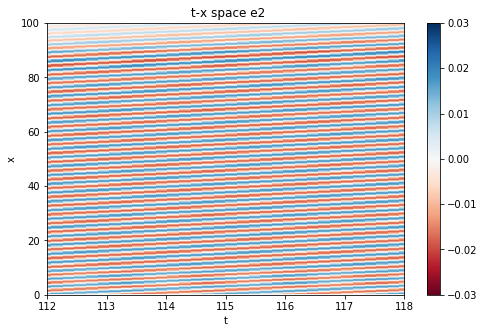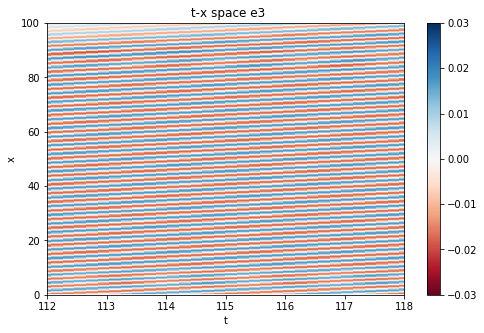Next we plot dots of the values of the electric field on an $E_2$ vs. $E_3$ plot. Each dot corresponds to a different time. The colors correspond to different values of $x_1$. Blue is for an $x_1$ in the vacuum before the EM wave enters the plasma, red is for an $x_1$ in the plasma, and green is for an $x_1$ in the vacuum after the wave exits the plasma.

• the blue line is the polarization as a function of time at $x_1=2.0$
• the red line is the polarization as a function of time at $x_1=48.0$
• the green line is the polarization as a function of time at $x_1=96.0$
dirname = 'xmode-b05-flat-2.2'

from h5_utilities import *
import matplotlib.pyplot as plt

nt = e2.data.shape
tmax=e2.axes.axis_max
dt=tmax/(nt)
time_axis=np.arange(0,tmax,dt)

plt.scatter(e2.data[:,10],e3.data[:,10],c='b',label='in vacuum at x1=2',s=2)
plt.scatter(e2.data[:,240],e3.data[:,240],c='r',label='in plasma at x1=48',s=2)
plt.scatter(e2.data[:,480],e3.data[:,480],c='green',label='in vacuum at x1=96',s=2)

plt.legend()
plt.xlabel('$E_2$',fontsize=18)
plt.ylabel('$E_3$',fontsize=18)
plt.show()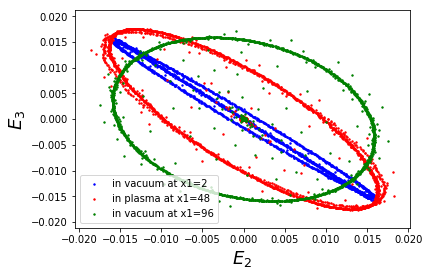Can you explain this plot? The blue dots should form a straight line, but some of the signal is reflected which makes it more complicated. Understanding this result will be helpful for the final exam.